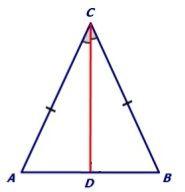# Prove that angles opposite to equal sides of an isosceles triangle are equal.

Given:
An isosceles triangle ABC where AC = BC.
To prove:
∠CAB = ∠CBA.
Construction:
CD, the bisector of ∠ACB.Proof:
Now in ∆ACD and ∆BCD we have,
AC = BC (Given)
∠ACD = ∠BCD (By construction)
CD = CD (Common to both)
Thus, ∆ACD ≅∆BCD (By SAS congruence criterion)
So, ∠CAB = ∠CBA (By CPCT)
Hence proved.(3)(0)